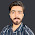## Saturday, 23 December 2017

### Python - Function

Most applications weather they written in python or some other programming languages broke up in smaller block of codes known as functions. So in python there is no difference in functions.

### Default / Simple Function

```#!/usr/bin/python3

# working with functions

#define a function
def fun1():
print("This is a function")
```

In python we define a function with def keyword and given a name fun1. This function does not take any argument or parameter. Here colon indicates that you are starting the scope of that function. And in the scope of above function we have written print statement. Here you will notice that we have written the print statement within the scope of the function. You will also notice that we have intended the print statement as compare to the function scope, its mean this print statement is the part of the function.

Now it is the time to call that function. To call the above function we use below code snippet.

```def main():
fun1()
print(fun1())
print(fun1)
if __name__ == "__main__": main()`       `
```

In line no 11 you can see that when we call fun1() it prints out "This is a function". In line no 12 when this statement runs "print(fun1())" actually first fun1() will be called and it prints out, and after that print(fun1()) will be executed and this time our function return nothing (fun1 have no return value) that's why it prints out "None". In line no 13 we have print only fun1 with our parenthesis. Here you will notice that their print out is some hexadecimal numbers this is happening because everything in python is an object and function is also an object and have some value. In this case it is an object, a function object. Here print command is printing out the value of fun1 object.

Now you know that how python treats object.

### Parameterized Function

Parameterized functions take arguments. Below code snippet shows how to declare these types of functions in python.

```#!/usr/bin/python3

# working with functions

#define a parameterize function
def parameter_fun(arg1,arg2):
print(arg1, " ", arg2)`      `
```

In above code snippet we define a parameterize function that take two arguments (arg1 and arg2). And inside the function scope we have one print statement that will print the above arguments with a space separator.

The function call is same as we have been discussed in case of normal or default function. But the little difference is that in function call we pass two values or parameter. As shown below.

```def main():
parameter_fun(3,5)
print(parameter_fun(3,5))
if __name__ == "__main__": main()```
```
```

### Function with return value

In this example we will define a function that will return some value. For example here we are going to define a function that will return cubic value of a given number.

```#!/usr/bin/python3

# working with functions

#define a function that will return some value
def cube(given_value):
return given_value*given_value*given_value

def main():
print(cube(5))
if __name__ == "__main__": main() ```
```
```

In above code snippet we define a function named cube(), this function take one parameter or argument of type number or integer. And inside the function scope we return three times of that number. So this function return cube of the given number.

I hope you will understand functions in python. Functions can be used to code reusability. For example you have a function that will calculate some complex problems, algorithms and get values from the database and return the value. Now you can use this function inside your program multiple times whenever you need. You don't need to write code multiple times just define function and call that function.

#### 1 comment:

1.Very nice blog. keep it up